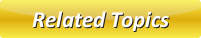# Inferential Statistics

Inferential Statistics is a process that is applied by the researchers for generalizing and to infer the observations which is done with samples to the bigger population from which they were chosen.## Difference between Descriptive and Inferential Statistics

1. The descriptive statistics is basically helps in giving the data about a set of information which were collected. Whereas, Inferential statistics is basically used for making comparison and predicting  a big population with the help of information collected from the small population of that part. Therefore, inferential statistics aids in generalizing more that the data in which we don't get it on descriptive statistics.

2. The discrete data contains whole numbers & normally its the count of things (For instance, counting number of fish in the fish pond where half or quarter kind of quantities don't play any roles.

3. The measured data in comparison to discrete data could be continuous and possess real values in them. (For instance, children playing in play ground can be a measured data and as the children could play any amount of time their playing habits would probably be the multiples of 60 minutes.)

4. The numerical data would always possess numbers.

5. The categorical data would possess words. (For instance, a list of cars in a car showroom would contains a category of companies make like Honda, Lamborghini, Mitsubishi etc.)

Latest Articles

Average Acceleration Calculator

Average acceleration is the object's change in speed for a specific given time period. ...

Free Fall Calculator

When an object falls into the ground due to planet's own gravitational force is known a...

Permutation

In Mathematics, the permutation can be explained as the arrangement of objects in a particular order. It is an ordered...

Perimeter of Rectangle

A rectangle can be explained as a 4-sided quadrilateral which contains equal opposite sides. In a rectangle

Perimeter of Triangle

A three sided polygon which has three vertices and three angles is called a triangle. Equilateral triangle...